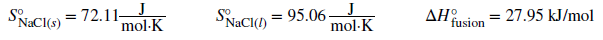# Problem: By calculating ΔSuniv at each temperature, determine if the melting of 1 mole of NaCl(s) is spontaneous at 500 °C and at 700 °C.What assumptions are made about the thermodynamic information (entropy and enthalpy values) used to solve this problem?

⚠️Our tutors found the solution shown to be helpful for the problem you're searching for. We don't have the exact solution yet.

###### Problem Details

By calculating ΔSuniv at each temperature, determine if the melting of 1 mole of NaCl(s) is spontaneous at 500 °C and at 700 °C.What assumptions are made about the thermodynamic information (entropy and enthalpy values) used to solve this problem?